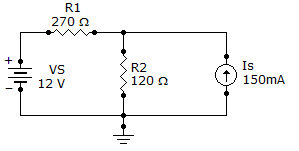# Electrical Engineering - Circuit Theorems and Conversions - Discussion

### Discussion :: Circuit Theorems and Conversions - General Questions (Q.No.5)

5.

Find the current through R2 of the given circuit.[A]. 30.7 mA [B]. 104 mA [C]. 74 mA [D]. 134 mA

Explanation:

No answer description available for this question.

 Pk Pandey said: (Feb 8, 2011) I make use of kcl but not able to get ans.

 Deepa said: (Jul 15, 2011) Do oc of current and apply ohms law so current=31ma and sc voltage source apply current divider rule then you obtain current=103.84ma add both you get 134ma(approx).

 Mahesh Kumar Tanwar said: (Nov 9, 2011) Why we can't apply kcl. I think kcl make it eassy.

 M.V.Krishna/Palvoncha said: (Dec 18, 2011) Use nodal analysis, you will get nearer value to option D.

 Archana said: (Aug 27, 2012) 0=V-12/270+V/120+150 V=16.15 current through R2 I=V/R I=16.15/120 =134mA

 Ruplal Rajak said: (Sep 4, 2012) Use of source transformation techniqu make will make the problem easy,120*0.15=18.00v will connected with series with 120 ohm resister and then apply kvl 120i+270i+18-12=-6/390

 Alwazzan said: (Jul 24, 2013) I1 = I2 - 0.150 ---- 1. v = 270I1 + 120I2 ---- 2. 12 = 270(I2-0.150) + 120I2 I2 = (12+270*0.150)/390 = 134 mA.

 Diksha said: (Nov 21, 2013) By using nodal analysis, we can easily get the final result 134 mA.

 Omprakash Jatav said: (Jan 27, 2014) By using kvl, we can solve the easily final result 134.12.

 Sandeep said: (Feb 6, 2014) By applying nodal analysis, instead of -150 why +150 is represented.

 Amke Naveen said: (Dec 5, 2014) Use super position theorem first consider dc voltage source open current source. So i1 = 12/270+120. Then current source i2 = 150m*270/270+120. I = i1+i2.

 Dusmanta Rohiodas said: (Apr 13, 2015) 0 = V-12/270 + V/120 + 150. V = 16.15. Current through R2. I = V/R. = 16.15/120. = 134 mA.

 Vignesh said: (Nov 9, 2015) Please tell this problem.

 Jagadesh said: (Feb 23, 2016) By applying nodal analysis. Current entering = Current leaving. Assume the 120 ohm branch node as v. 150 mA = ((V - 12)/270) + (V/120). 0.15 = (13 V - 48)/1080. 162 = 13V - 48. 13 V = 210. V = 210/13 V. Current in 120 ohm branch = V/120. = 210/(13*120). = 7/(13*4) = 7/52 = 0.134. = 134 mA.

 Basireddy said: (Feb 9, 2017) Write loop equation. 270(i1)+120(i1+i2)=12V ..........eqn-1 And i2=150mA. Now solving eqn-1. 270(i1)+120(i1)+(120*150mA) = 12V 390(i1)+18V = 12V 390(i1) =12V-18V 390(i1) = -6V i1 = -6V/390 i1 = -15.38mA Now current flowing through R2 is (i1 + i2). Therefore, -15.38mA + 150mA = 134.62mA.

 D.Mahammad Ali said: (Mar 13, 2017) By nodal annalysis first find node voltage then find the current.

 Md, Rayhan Uddin said: (Jul 12, 2017) 0 = V-12/270 + V/120 " 0.15, =>(4V-48+9V-162)=0, =>13V=210, =>V=210/13, =>V=16.15V, Since, IR2=16.15/120, =134mA.

 Fayaz said: (Dec 9, 2017) 16.15/120=.134amp. 134/1000=1.34ma.

 Anon said: (Dec 19, 2019) By nodal analysis we will arrived to this equation; (V-12)/270 + V/120 -150mA =0 . V=16.15V. Hence, I2=16.15/120. I2=1.346mA.